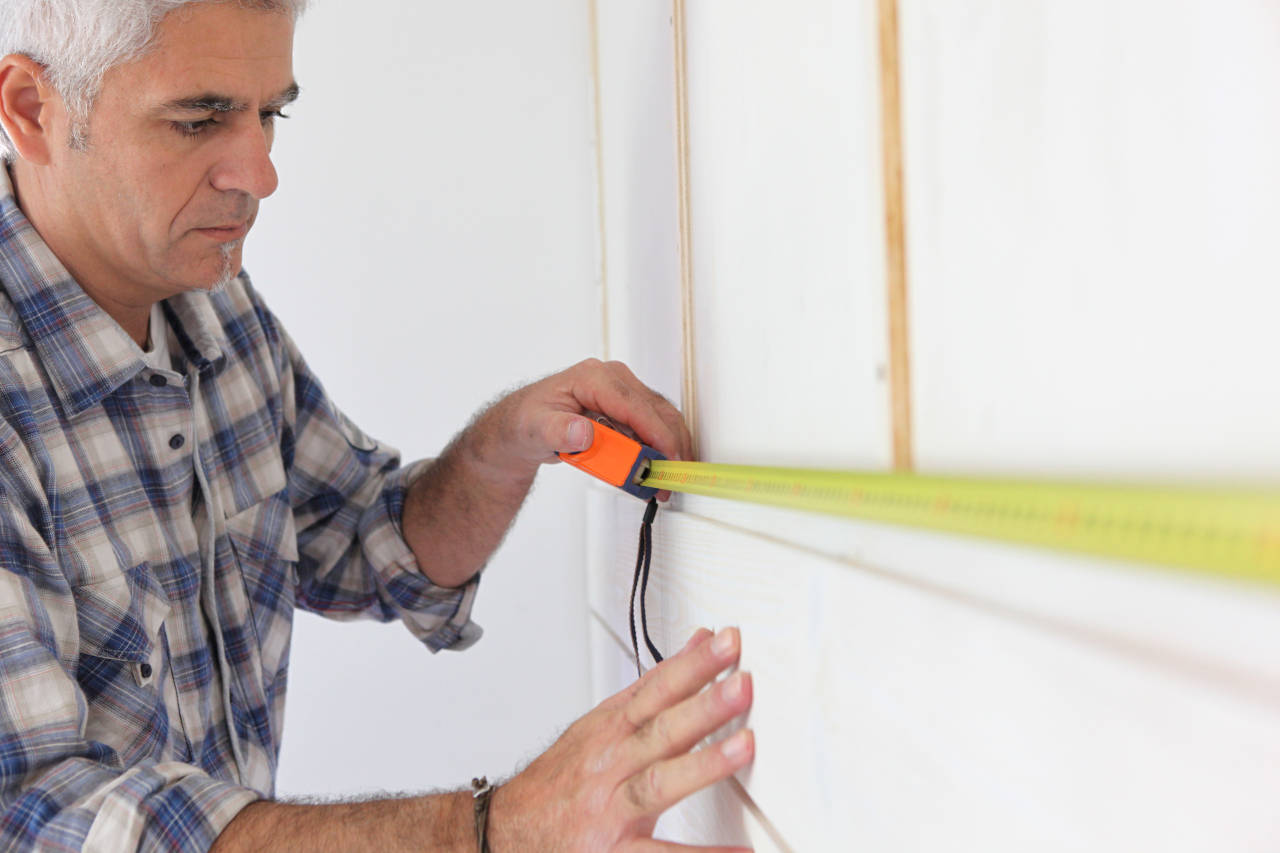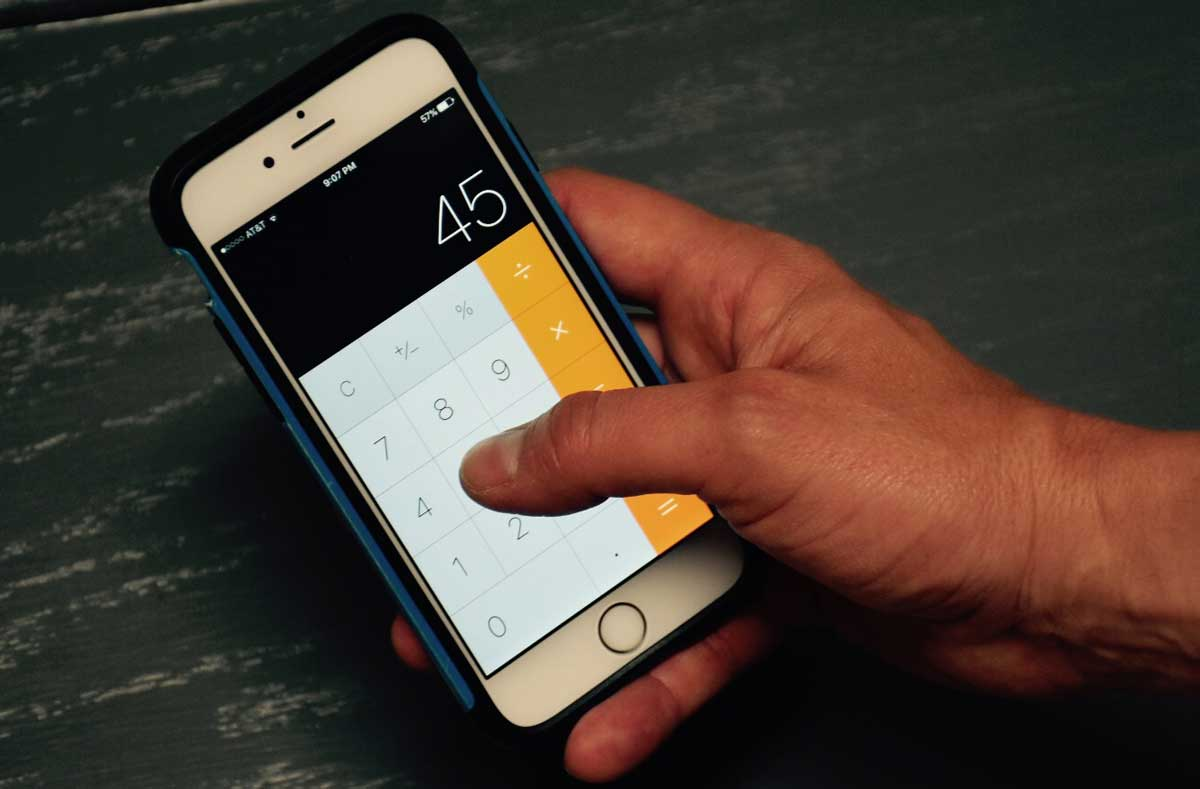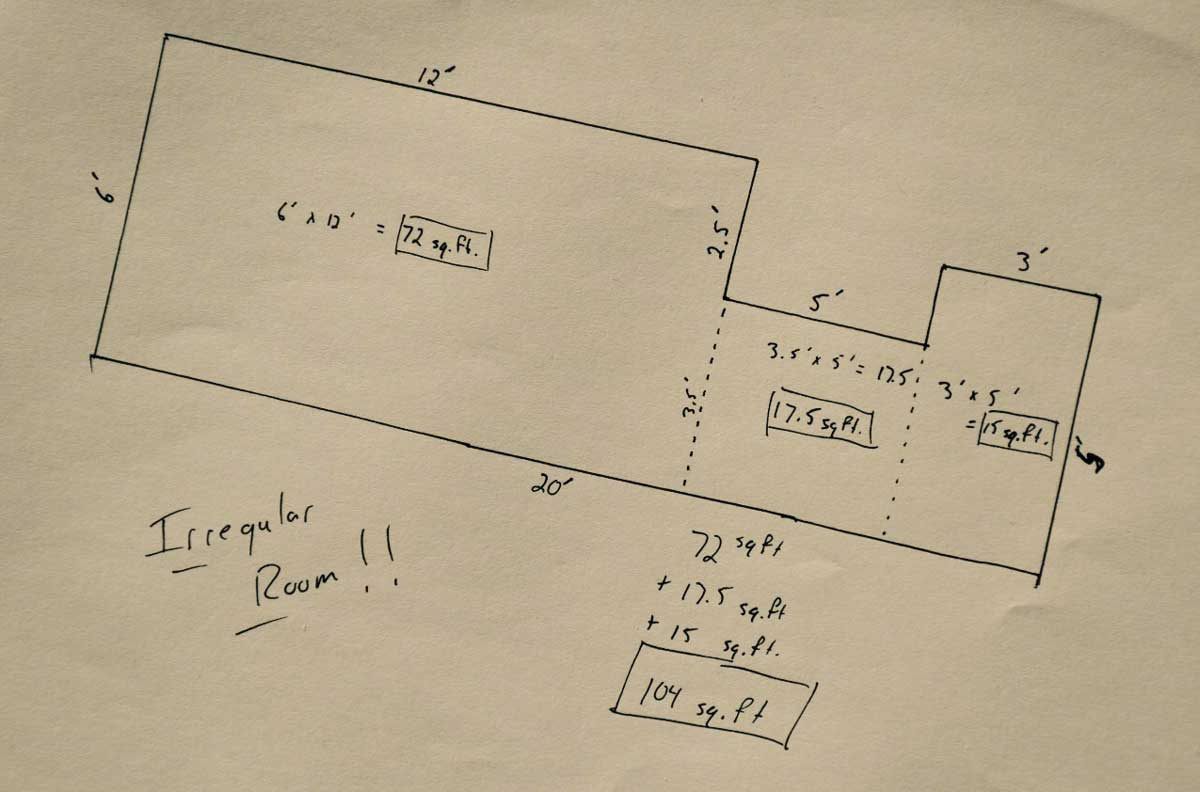# How to Measure a Room for Home Improvement ProjectUnderstanding how to properly measure a room can save both time and money. Don’t get caught underestimating the amount of material that is needed for a project.

Making unnecessary trips to a store or supplier can be a hassle and waste of time and delivery fees can add to project costs. Special orders for material that can take days or weeks to arrive can be even more problematic.

On the flip-side, there is no need to overspend and end up with excess material sitting around collecting dust.

So it’s worth taking a few minutes to correctly measure a room before ordering to eliminate this prevent headache.

The tools to correctly measure a room are pretty basic. All you’ll need is a tape measure and a calculator.

## How to Measure a Rectangular Room

The principles of measuring a square or rectangular room are simple, requiring just two steps.

### Step One: Take Measurements

Choose two perpendicular walls (walls that intersect to form a corner) and measure the length of each using a tape measure.### Step Two: Calculate Square Feet

Use your calculator to multiply the two measurements. For example, a square or rectangular room that has one wall that measures and 8 feet long and a perpendicular wall that is 10 feet long will result in the follow equation.

8 ft × 10 ft = 80 sq ft

Thus, this room is 80 square feet.There are also online square footage measurement tools that can multiply these measurements.

Got it? That may seem basic, but what if the room isn’t square or rectangular?

## How to Measure Irregular Rooms

Irregularly shaped rooms can prove to be a bit of challenge. It might seem counter intuitive to figure the square footage of a room that isn’t square, but just as before, this can be done in a few easy steps.

### Step One: Split up the Room

The first step is to divide the room up into multiple square sections. Separate complex areas or nooks into separate areas that can be measured separately from the rest of the room.

### Step Two: Calculate the Area of Each Section

When the room has been divided up into several imaginary square sections, figure the square footage of each one separately using the method above.

It might be easiest to sketch a simple floor outline of the room. Then, cross-section the room and identify the square sections.

Next, measure the edges of each each square section and calculate the square footage of each one just as before. Take note of the results of each section, you’ll need that in a moment.### Step Three: Add the Areas Together

Once to total of each section has been figured, they can be added together. Add the area of each section to the total until you’ve accounted for all of the floor space on the sketch.

These steps are a great way to estimate how much material would be needed to complete a flooring project, for instance new carpet or hardwood.

However, this method of measuring a room will not work to estimate the area of walls or wall coverings. Estimating for drywall, paneling, paint or wallpaper will require a slightly different method of measuring.

Measuring the square footage of the walls in a room utilizes the same principles, but in a slightly different way.

## How to Measure Walls for Paint or Wallpaper

To accurately measure a room for wall coverings we will use the length of the wall, and the height to the ceiling. The formula will be similar to before.

### Step One: Take Measurements

Start by measuring the length of the wall and the height of the ceiling using a tape measure. If you have multiple walls, then do this for each wall surface.

### Step Two: Calculate the Area of Each Wall

Next, use a calculator to multiply the length and height, just like before. If, for example, the wall measures 12 feet long and 8 feet high, use the calculator to figure 12 feet × 8 feet and see that the wall is 96 square feet.

### Step Three: Add the Wall Areas Together

Repeat this process for each segment of wall in a room to accurately measure a room for any type of wall-coverings. Add the areas of each wall together to get the total square footage of all the walls.

### Step Four: Subtract Doors and Window Openings

When measuring for some materials such as paint it might be important to subtract surfaces that will not be covered like doors or windows. Note that when measuring for some material accounting for doors and windows may not be necessary and this step can be skipped.

As you make your way around your room, measuring the square footage of each segment, make sure that you note any doors and windows. Measure the length and width of each one, then multiply to find the area. Finally, subtract each door and window area from the total.

One quick tip: our wallpaper and paint calculators can help estimate wall covering materials after you’ve measured your walls and simplify this whole process, so it might be worth checking them out.